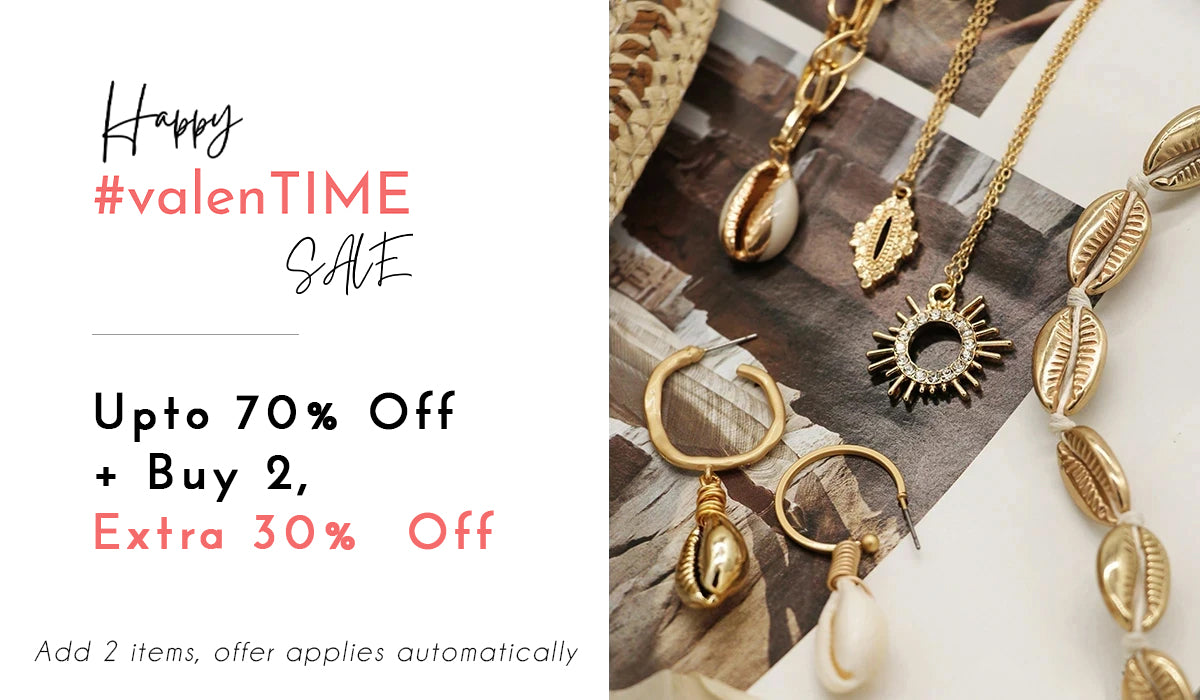# Everything under 999• Sort by

• Shop by Color

• Shop by Style

• Shop by Type

SALE

Rs. 999.00
Rs. 1,799.00
SALE

Rs. 999.00
Rs. 1,599.00
SALE

Rs. 999.00
Rs. 1,799.00
SALE

Rs. 999.00
Rs. 2,199.00
SALE

Rs. 999.00
Rs. 2,199.00
SALE

Rs. 999.00
Rs. 2,199.00
SALE

Rs. 999.00
Rs. 1,799.00
SALE

Rs. 999.00
Rs. 1,799.00
SALE

Rs. 999.00
Rs. 1,899.00
SALE

Rs. 999.00
Rs. 1,899.00
SALE

Rs. 999.00
Rs. 1,899.00
SALE

Rs. 999.00
Rs. 1,799.00
SALE

Rs. 999.00
Rs. 2,099.00
SALE

Rs. 999.00
Rs. 2,099.00
SALE

Rs. 999.00
Rs. 1,999.00
SALE

Rs. 999.00
Rs. 1,899.00
SALE

Rs. 999.00
Rs. 1,699.00
SALE

Rs. 979.00
Rs. 2,449.00
SALE

Rs. 979.00
Rs. 2,449.00
SALE

Rs. 950.00
Rs. 1,899.00
SALE

Rs. 950.00
Rs. 1,899.00
SALE

Rs. 950.00
Rs. 1,899.00
SALE

Rs. 950.00
Rs. 1,899.00
SALE

Rs. 950.00
Rs. 1,899.00
SALE

Rs. 950.00
Rs. 1,899.00
SALE

Rs. 950.00
Rs. 1,899.00
SALE

Rs. 950.00
Rs. 1,899.00
SALE

Rs. 950.00
Rs. 1,899.00
SALE

Rs. 950.00
Rs. 1,899.00
SALE

Rs. 950.00
Rs. 1,899.00
SALE

Rs. 950.00
Rs. 1,899.00
SALE

Rs. 950.00
Rs. 1,899.00
SALE

Rs. 949.00
Rs. 1,599.00
SALE

Rs. 900.00
Rs. 1,799.00
SALE

Rs. 900.00
Rs. 1,799.00
SALE

Rs. 900.00
Rs. 1,799.00
SALE

Rs. 900.00
Rs. 1,799.00
SALE

Rs. 900.00
Rs. 1,799.00
SALE

Rs. 900.00
Rs. 1,799.00
SALE

Rs. 900.00
Rs. 1,799.00
SALE

Rs. 900.00
Rs. 1,799.00
SALE

Rs. 900.00
Rs. 1,799.00
SALE

Rs. 900.00
Rs. 1,799.00
SALE

Rs. 900.00
Rs. 1,799.00
SALE

Rs. 900.00
Rs. 1,799.00
SALE

Rs. 900.00
Rs. 1,799.00
SALE

Rs. 900.00
Rs. 1,799.00
SALE